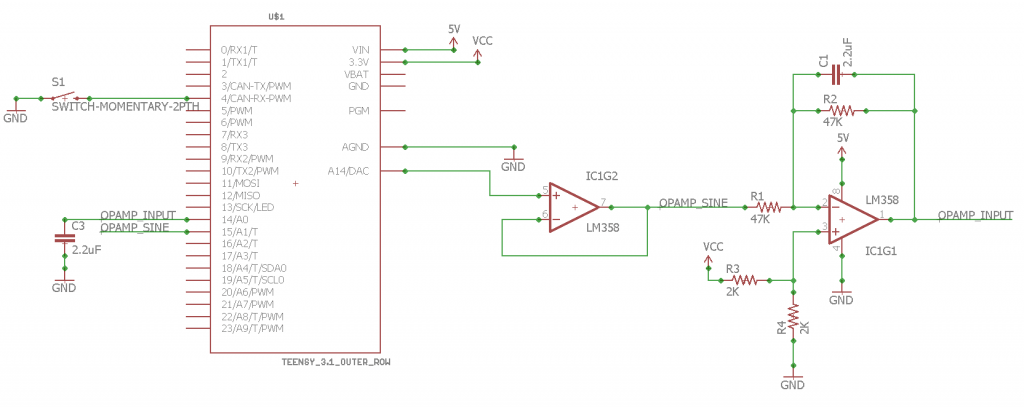Op-Amp Experimentation 1: Op-Amp Basics

Op-Amp Experimentation 2: Basic Circuit Math

Op-Amp Experimentation 3: Op-Amp Applications

Op-Amp Experimentation 4: From Ideal to Real

Op-Amp Experimentation 5: Integrator

Op-Amp Experimentation 6: Differentiator

Op Amp Experimentation 7: PID Controller – coming soon

Okay, let’s test some of this out. For the record, I am using Visual Studio 2015 and a Teensy 3.2 for these tests. The first thing I want to try out is the integrator.The equation for this is:Here is the circuit that I made for doing some integration:I had the A14 pin on the Teensy write a simple sine wave, and recorded the input. Here is the resulting output:

As you can see, if the OUTPUT is a sine wave, the INPUT is the integration of that sine wave. It works! Here is the Arduino code I made:

/******************************
Op Amp Integration
by Taylor Schweizer

This program is meant to be
a way of testing an op-amp
integration circuit.
See my website learn-cnc.com
for more details on the circuit.
******************************/

//Pin declarations!
//PWM_Sense - analog pin for measuring PWM pin
//OPAMP_Pin - analog pin for measuring op-amp output
//PWM pin - ...pwm pin
//Start_Interrupt - pin used for starting a test
int SINE_INPUT = 14;
int OPAMP_INPUT = 15;
int INTERRUPT_PIN = 4;

void setup()
{
//Options for the Teensy 3.2, not sure if it'll work with your microcontroller
analogReference(DEFAULT);

//Begin serial, print some information
Serial.begin(9600);

//Setup pins
pinMode(SINE_INPUT, INPUT);
pinMode(OPAMP_INPUT, INPUT);
pinMode(A14, OUTPUT);
pinMode(INTERRUPT_PIN, INPUT_PULLUP);
analogWrite(A14, 128);
}

void loop()
{
//Basically just a simple psuedo interrupt code
delay(1000);
startTest();
}

}

void startTest() {

long startTime = micros();
//Begin a sine wave so the op-amp can reach a stable value
//It kept climbing when I first tried this out, so this just
//lets the signal stabilize
for (int j = 1; j < 100; j++) {
for (float i = 0.01; i < 6.28; i = i + 0.01) {
analogWrite(A14, (sin(i) * 100.0 + 128.0));
}
}

//Here is the actual test. Open the Serial Monitor,
//And push the button in the schematic to begin the test
startTime = micros();
for (int j = 1; j < 10; j++) {
for (float i = 0.01; i < 6.28; i = i + 0.001) {
analogWrite(A14, (sin(i) * 100.0 + 128.0));
Serial.print(micros() - startTime);
Serial.print("\t");